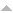## Publications

Export 3 results:
Sort by: Author [ Title] Type Year
L
Chaari, S., F. Cipriano, and H. Ouerdiane. "Large deviation properties of solutions of nonlinear stochastic convolution equations." Adv. Theor. Appl. Math.. 2 (2007): 1-14. Abstract

n/a

Chaari, S., F. Cipriano, and H. Ouerdiane. "Large deviations for infinite dimensional analytical distributions." Adv. Theor. Appl. Math.. 1 (2006): 173-187. Abstract

n/a

Cipriano, Fernanda, and Tiago Costa. "A large deviations principle for stochastic flows of viscous fluids." JOURNAL OF DIFFERENTIAL EQUATIONS. 264 (2018): 5070-5108. Abstract

{We study the well-posedness of a stochastic differential equation on the two dimensional torus T-2, driven by an infinite dimensional Wiener process with drift in the Sobolev space L-2 (0, T; H-1 (T-2)). The solution corresponds to a stochastic Lagrangian flow in the sense of DiPerna Lions. By taking into account that the motion of a viscous incompressible fluid on the torus can be described through a suitable stochastic differential equation of the previous type, we study the inviscid limit. By establishing a large deviations principle, we show that, as the viscosity goes to zero, the Lagrangian stochastic Navier-Stokesflow approaches the Euler deterministic Lagrangian flow with an exponential rate function. (c) 2018 Elsevier Inc. All rights reserved.}